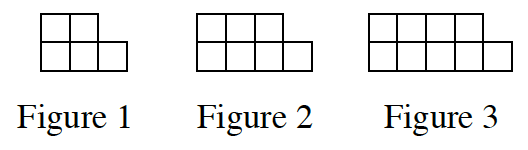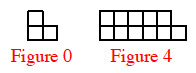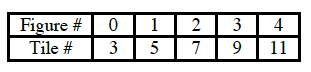Home > ACC7 > Chapter cc34 > Lesson cc34.1.3 > Problem4-25

4-25.

Two of the connections in your Representations of Patterns Web are pattern → table and pattern → rule. Practice these connections as you answer the questions below.  Homework Help ✎

1. On graph paper, draw Figure 0 and Figure 4 for the pattern at right.What is the growth between the number of tiles in each figure?2. Represent the number of tiles in each figure with a table.3. Represent the number of tiles in each figure with an algebraic rule.

What is the growth between the number of tiles in each figure?

What is the starting amount?

$y=2x+3$